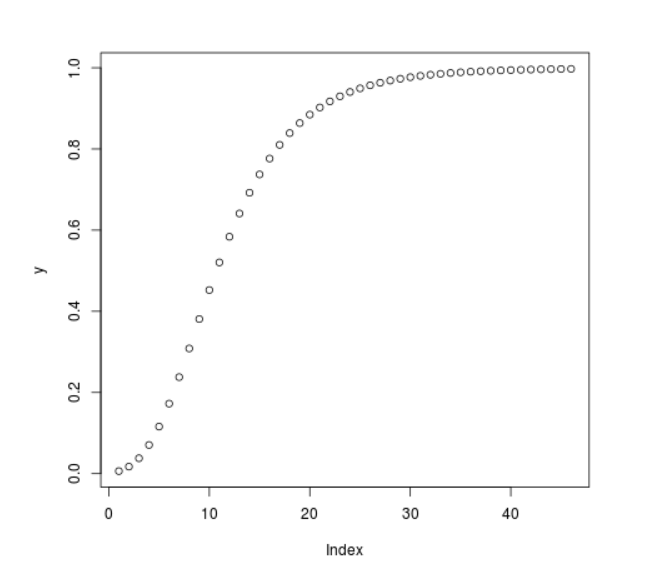GFG App
Open AppBrowser
Continue

# Compute the value of CDF over Studentized Range Distribution in R Programming – ptukey() Function

`ptukey()` function in R Language is used to compute the value of Cumulative Distribution Function(CDF) over Studentized Range for a sequence of numeric values.

Syntax: ptukey(x, nmeans, df)

Parameters:
x: Numeric Vector
nmeans: Number of means
df: Degree of Freedom

Example 1:

 `# R Program to compute the value of ` `# CDF for Studentized Range ` ` `  `# Creating a sequence of Numeric values ` `x <``-` `seq(``1``, ``10``, by ``=` `1``) ` ` `  `# Calling ptukey() Function ` `y <``-` `ptukey(x, nmeans ``=` `7``, df ``=` `5``) ` `y `

Output:

```  0.01416051 0.22099429 0.54676395 0.76823006 0.88284988 0.93871676
 0.96637587 0.98063547 0.98833593 0.99268670
```

Example 2:

 `# R Program to compute the value of ` `# CDF for Studentized Range ` ` `  `# Creating a sequence of Numeric values ` `x <``-` `seq(``1``, ``10``, by ``=` `0.2``) ` ` `  `# Calling ptukey() Function ` `y <``-` `ptukey(x, nmeans ``=` `8``, df ``=` `7``) ` ` `  `# Plot a graph ` `plot(y) `

Output:My Personal Notes arrow_drop_up